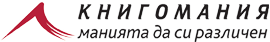Език
Валута

#Кошница

## ESSENTIAL MATLAB FOR ENGINEERS AND SCIENTISTS. 3

Няма наличност
ISBN
9780750684170
65,00 лв.
Description The essential guide to MATLAB as a problem solving tool This text...
Автор B.Hahn, D.Valentine 424 английски 2007 ELSEVIER 26888417 9780750684170 Компютри и графичен дизайн

Description The essential guide to MATLAB as a problem solving tool This text presents MATLAB both as a mathematical tool and a programming language, giving a concise and easy to master introduction to its potential and power. Stressing the importance of a structured approach to problem solving, the text gives a step-by-step method for program design and algorithm development. The fundamentals of MATLAB are illustrated throughout with many examples from a wide range of familiar scientific and engineering areas, as well as from everyday life. Features: – Includes MATLAB Version 7.2, Release 2006a – Numerous simple exercises provide hands-on learning of MATLABs functions – A new chapter on dynamical systems shows how a structured approach is used to solve more complex problems. – Common errors and pitfalls highlighted – Concise introduction to useful topics for solving problems in later engineering and science courses: vectors as arrays, arrays of characters, GUIs, advanced graphics, simulation and numerical methods – Text and graphics in four colour – Extensive instructor support Essential MATLAB for Engineers and Scientists is an ideal textbook for a first course on MATLAB or an engineering problem solving course using MATLAB, as well as a self-learning tutorial for students and professionals expected to learn and apply MATLAB for themselves. Additional material is available for lecturers only
Напишете вашето мнение
Вие оценявате:ESSENTIAL MATLAB FOR ENGINEERS AND SCIENTISTS. 3
Нови книги от тази подкатегория
Бестселъри

Knigomania.bg използва Бисквитки. Използвайки този уебсайт, вие приемате нашите Общи Условия и Политика за поверителност.